# Java Program to Find Largest and Smallest Number in an Array

In this tutorial you will learn about the Java Program to Find Largest and Smallest Number in an Array and its application with practical example.

In this tutorial, we will learn to create a Java program that will Find Largest and Smallest number in an array using Java programming.

## Prerequisites

Before starting with this tutorial we assume that you are best aware of the following Java programming topics:

• Operators.
• looping statements.
• Basic input/output.
• Basic Java programming
• For Loop.
• Array.

## What is array?

An Array is a collection variable elements  that can store multiple values in a single variable of same type.In Computer Science,an array is a data structure, or simply an array, is a data structure consisting of a collection of elements.

## Java Program to Find Largest and Smallest Number in an Array

In this program we will Find Largest and smallest Array elements. First of all we declared and initialized the required variables. then we would prompt user to input elements of an Array Later we will find largest and Smallest element in an Array.

## Output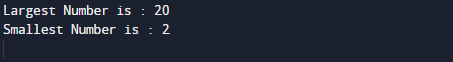In the above program, we have first declared and initialized a set variables required in the program.

• num[] = it will hold entered elements.
• large =it will hold largest Element.
• small=it will hold smallest elements.
• i= for iteration.

In our program, we will use 10 values in an array , after adding these values we will find the
smallest and largest elements of an array.
First of all we declare variables and initialize array with elements and  on variable small and large we will assign the first elements of an array.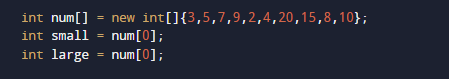Next within the loop we will check each elements with the large and small variable,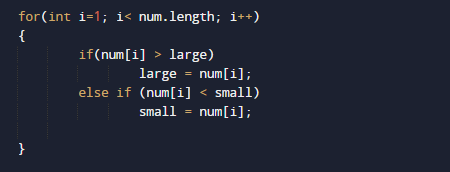Here we will compare each elements of an Array to large variable which hold the first value of an array , if any element of an array found greater than large  then the value we will swap that value to large. As shown in image below.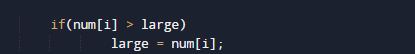This process will continues same as above here we compare condition fro smallest where we check if value of small is greater than any elements of an array we replace that to small. As shown image below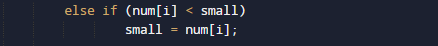At the end we have largest value in large variable and smallest in small variable.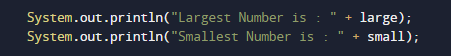then finally we will print the result at last as shown above.

In this tutorial we have learn about the Java Program to Find Largest and Smallest Number in an Array and its application with practical example. I hope you will like this tutorial.